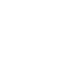# Feb 24 ACT & SAT Question of the Day

http://sat.collegeboard.org/practice/sat-question-of-the-day?src=R&questionId=20130224 (This link takes you to today’s question. If you use my archive, you will see the question related to my SAT explanation for that date.)

The answer is E.  I think a robot writes the SAT math explanations!  They always sound the same and don’t tell you much about how to improve your score.  Let’s really take a look at this question and learn a few things about the test so that we can use this question to become better test takers.

The SAT and ACT test writers love to describe lines on the coordinate grid without giving you a diagram.  When they do that, just draw one that is based on the informatioon (Pillar II).  When I did so with this question, I got a line with zero slope that intercepted the y-axis at +4.  You’ve probably already been introduced to the slope-intercept equation.  If not, take a look at DVD #8 or my website online course.  (y=mx +b where m is the slope and b is the y-intercept.) The slope in this case is zero and 0x (substituting for mx) in the equation means there’s no x in the equation.  +4 is substituted for b which leaves answer E.

What’s my tip?  Anytime you see a  question about a line on the grid for which you are given information about slope and the y-intercept, just use the slope-intercept equation.  This question type is relatively common and fairly simple if you see the signal in the question about what to do: slope and y-intercept.

Let’s take a look at the ACT question.

http://www.act.org/qotd/ (The ACT staff does not put a date on their questions so if you click on an archived blog, you’ll get today’s question and the old explanation. Sorry. The SAT staff has dated their questions; so, the archive is helpful. The ACT folks simply don’t do that.)

The answer is B.  The ACT explanation is fine.  Take a look at line 17 and you’ll see the answer.  In addition, let me use this question as an example to explain  something about the SAT and ACT tests that you should know.  See how this is a detail question?  You will very rarely see those on the SAT test.  They are extraordinarily rare.  That’s because the SAT folks give you line references for over 80% of the questions and this would be an very easy question if the question had said “in lines 16-18.”  The ACT folks aren’t so generous with their line references and so the challenge becomes finding the answer, not reading.  In this case, after you find the answer, you still have to deduce that the “difference” was that trial by battle was different in that it didn’t exist until after the Norman Conquest while the other two forms of trial existed prior to the Conquest.

My point is that when you take the ACT, you are going to spend a lot of time looking for answers since they don’t tell you where to look very often.  The SAT tells you where to look the majority of the time but they aren’t going to be simple detail questions.  Pick your poison!  You’ll need to try both tests to see which one works best for you.  (For most students the differences balance out!  You’ll most likely get an nearly equivalent score.  However there is a minority of students who will find one advantageous over the other.)  In other words, would you rather your trial be by compurgation, ordeal, or battle?! :-)